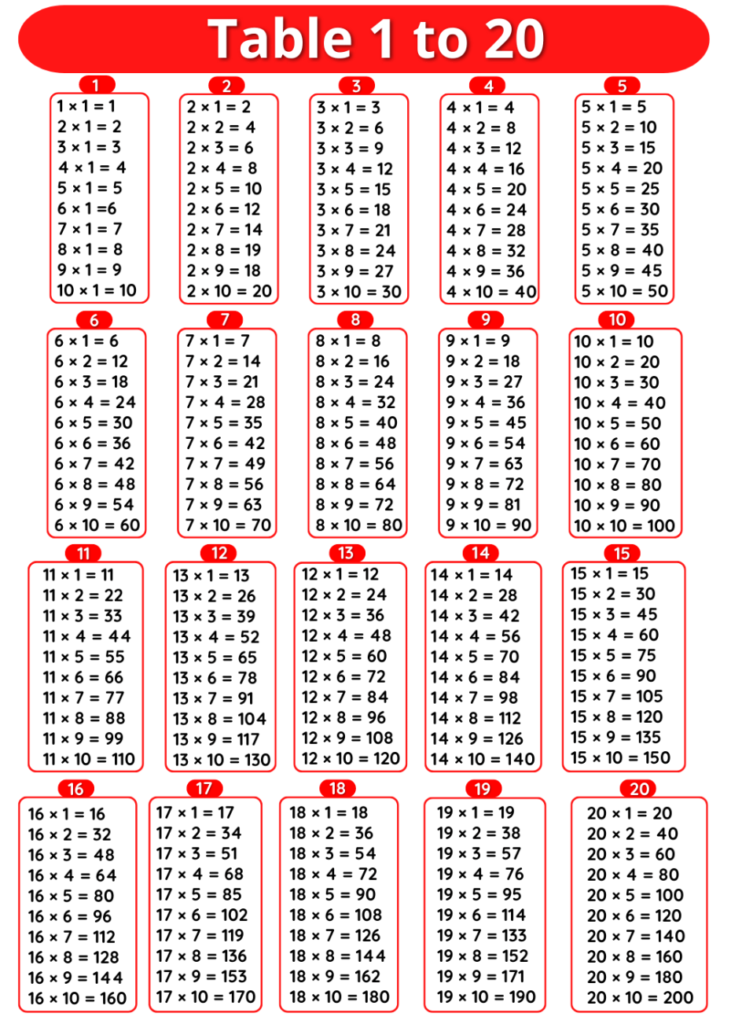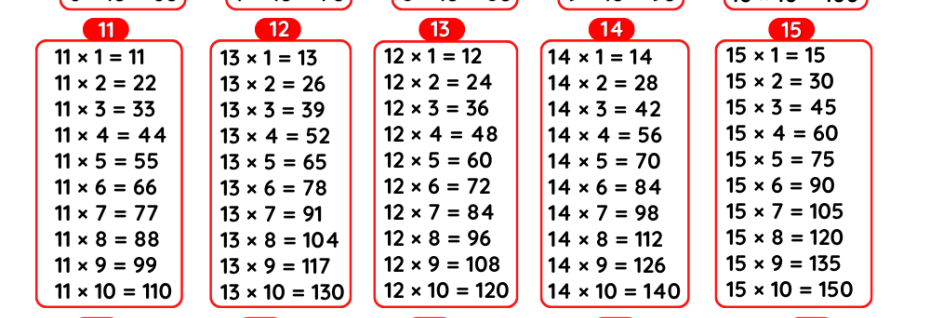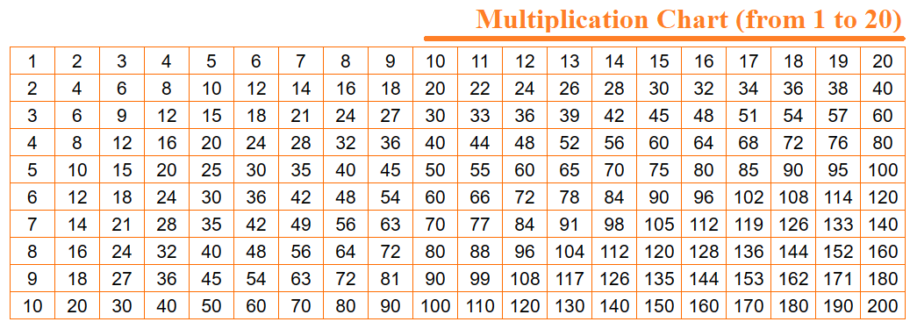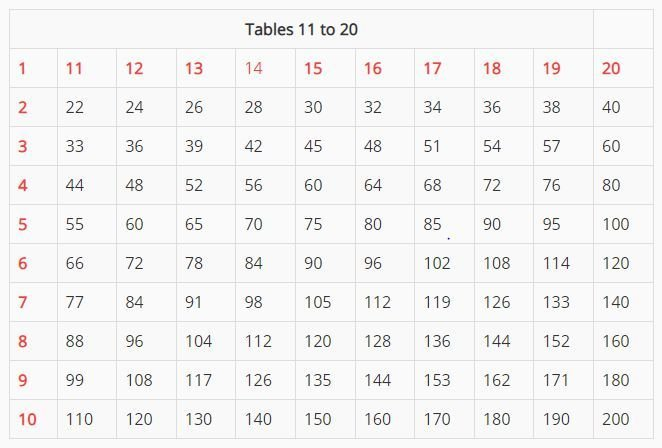# Tables 12 to 15, 12 se 15 tak table, Maths 12 to 15 tables Multiplication Chart

## Tables 12 to 15

Maths Multiplication Tables 12 to 15, Tables 12 to 15, 12 se 15 tak Table, 12 se lekar 15 tak Table, 12 to 15 Table Chart

### Tables 12 to 15

 Table of 12 Table of 13 Table of 14 Table of 15 12 ×‌ 1 = 12 13 ×‌ 1 = 13 14 ×‌ 1 = 14 15 ×‌ 1 = 15 12 ×‌ 2 = 24 13 ×‌ 2 = 26 14 ×‌ 2 = 28 15 ×‌ 2 = 30 12 ×‌ 3 = 36 13 ×‌ 3 = 39 14 ×‌ 3 = 42 15 ×‌ 3 = 45 12 ×‌ 4 = 48 13 ×‌ 4 = 52 14 ×‌ 4 = 56 15 ×‌ 4 = 60 12 ×‌ 5 = 60 13 ×‌ 5 = 65 14 ×‌ 5 = 70 15 ×‌ 5 = 75 12 ×‌ 6 = 72 13 ×‌ 6 = 78 14 ×‌ 6 = 84 15 ×‌ 6 = 90 12 ×‌ 7 = 84 13 ×‌ 7 = 91 14 ×‌ 7 = 98 15 ×‌ 7 = 105 12 ×‌ 8 = 96 13 ×‌ 8 = 104 14 ×‌ 8 = 112 15 ×‌ 8 = 120 12 ×‌ 9 = 108 13 ×‌ 9 = 117 14 ×‌ 9 = 126 15 ×‌ 9 = 135 12 ×‌ 10 = 120 13 ×‌ 10 = 130 14 ×‌ 10 = 140 15 ×‌ 10 = 150

### 12 se 15 tak Table, 12 se lekar 15 tak Table### Multiplication Tables 12 to 15### 12 to 15 Table chart

#### Tables 11 to 20